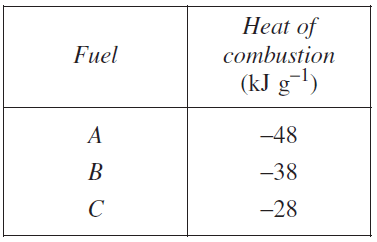Thermochemistry (2010 NSW HSC) 1)Write a balanced chemical equation for the complete combustion of 1-butanol. Solution (b) A student measured the heat of combustion of three different fuels. The results are shown in the table.The published value for the heat of combustion of 1-butanol is 2676 kJ mol−1. Which fuel from the table is likely to be 1-butanol? Justify your answer. Solution